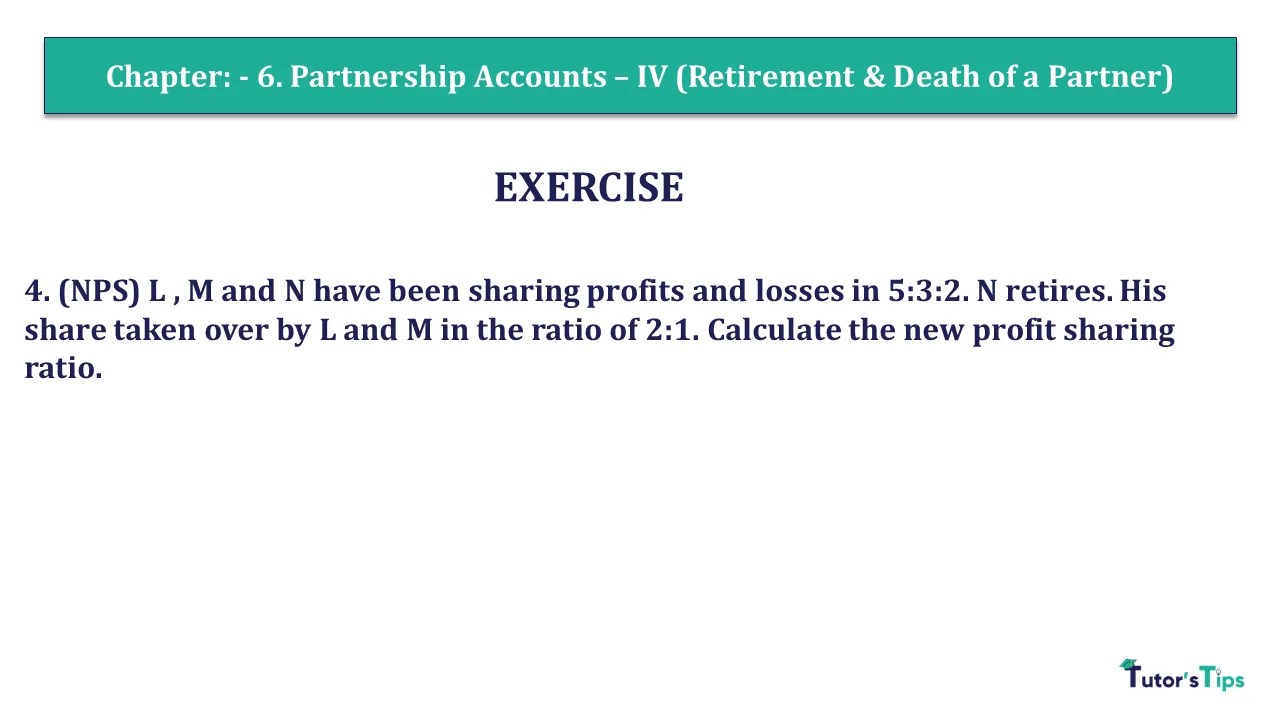# Question 04 Chapter 6 of +2 Part-1 – USHA Publication 12 Class Part – 1Q-4 - CH-6 - Usha +2 Book 2018 - Solution

Question 04 Chapter 6 of +2-Part-1

4. (NPS) L , M and N have been sharing profits and losses in 5:3:2. N retires. His share is taken over by L and M in the ratio of 2:1. Calculate the new profit sharing ratio.

## The solution of Question 04 Chapter 6 of +2 Part-1: –

Calculation of new Profit Sharing ratio

 N’s share in the profit of the firm = 2 10

Gaining ratio of L & M ( given ) = 2 : 1

 L’s gain = 2 x 2 3 10 = 4 30
 M’s gain = 1 x 2 3 10 = 2 30

New Share = Old share + Gain

 L’s New Share = 5 + 3 10 30 = 19 30
 M’s New Share = 3 x 2 10 30 = 11 30
 So, New Profit sharing Ratio = 19 : 11 30 30 = 19 : 11

Comment if you have any questions.

Also, Check out the solved question of previous Chapters: –